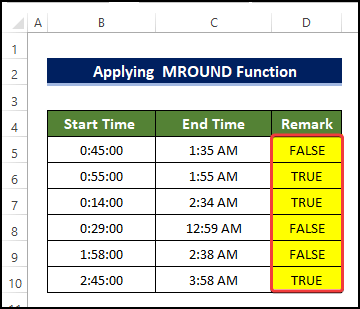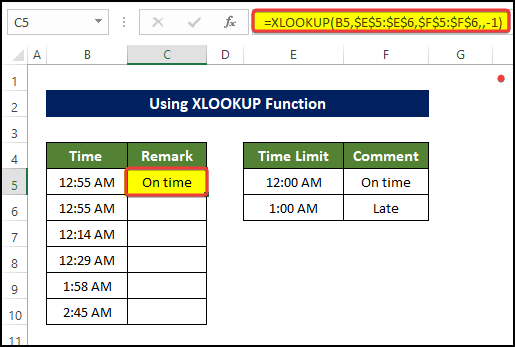# Return Expected Value If Time Is Greater Than 1 Hour in Excel

Get FREE Advanced Excel Exercises with Solutions!

Naturally, time is important for us, and so does whether we lag behind it or not. It is pretty normal to wonder if are going to lag behind from a certain submission time or not. If you are curious to know how you can determine if the time is greater than 1 hour or not in Excel, then this article may come in handy for you. In this article, we discuss how you know if the time is greater than 1 hour in Excel with elaborate explanations.

## How to Return Expected Value If Time Is Greater Than 1 Hour in Excel: 7 Suitable Examples

We are going to use the dataset, using which we demonstrate how we are going to determine whether the time period is greater than 1 hour or not. We are going to do this by using the remarks tags. we have the start time and the submission time of papers in the dataset for this.

### 1. Utilizing IF Function

If you want to have the flexibility of how much late or lag behind you are from a certain time, then using a cell reference value is the best way to approach this problem. We will use cell reference where we are going to put the amount of time in which we measure the time periods and whether they lag behind or not. We also going to use the IF function to help us in this method.

Steps

• Select cell C5 and enter the following formula:

`=IF(B5>\$E\$5, "Yes","No")`• Drag the Fill Handle to cell C10.
• Doing this will fill the range of cell C5:C10 with the remark of whether the time is greater than 1 hour or not in Excel.### 2. Applying  MROUND Function

The MROUND function is a classical function where we can get the rounded value of the first argument to the nearest multiple of the second argument. This function will allow us to get the verdict of whether the time periods mentioned are already greater than 1 hour or not.Steps

• Select cell C5 and enter the following formula:

`=MROUND(C5-B5, 1/86400) >= "1:00:00" * 1`• Drag the Fill Handle to cell D10
• Doing this will fill the range of cell D5:D10 with the remark of whether the time is greater than 1 hour or not.

###Read More: Return Value If Time Lies Between Multiple Time Ranges in Excel

### 3. Combining IF with TIME Function

We can combine functions like IF and TIME to create a formula that will be able to return remarks on if the time is greater than 1 hour or not.

Steps

• Select cell D5 and enter the following formula:

`=IF(C5 > B5 + TIME(1,0,0),"FAIL","PASS")`• Drag the Fill Handle to cell D10.
• Doing this will fill the range of cell D5:D10 with the remark of whether the time is greater than 1 hour or not in Excel.Formula Breakdown

➤ TIME(1,0,0)

• Here the TIME function denotes 1:00:00. The first argument denotes 1 hour, the second argument means 0 minutes and the third argument denotes 0 seconds.

➤ IF(C5 > B5 + TIME(1,0,0),”FAIL”,”PASS”)

• The first argument of the IF function is a logical argument. This argument checks whether the time value in cell C5 is greater than the time value in cell B5+return of the TIME function. If the logical argument is true, then the IF function will return “FAIL”, otherwise it will return “PASS”.

### 4. Calculate Difference in Hours

In this method, we will calculate the differences in time directly in seconds units, and then it will be converted to hour units. Using this we can determine whether the time difference is greater than 1 hour or not. We will take the help of the IF function also.

Steps

• Select cell D5 and enter the following formula

`=(C5-B5)*86400`• Drag the Fill Handle to cell D10.
• Doing this will fill the range of cell D5:D10 with the difference in time between submission time and start time in seconds.• Then in the cell E5, enter the following formula:

`=D5/3600`• Drag the Fill Handle to cell E10.
• Doing this will fill the range of cell E5:E10 with the difference in time between submission time and start time in hours.• Then select cell F5 and enter the following formula:

`=IF(E5>1,"Fail","Pass")`• Drag the Fill Handle to cell F10.
• Doing this will fill the range of cell F5:F10 with the remark of whether the time difference between the start time and submission time is greater than 1 hour or not.
• If the submission time is over 1 hour, then the remark will be “Pass” or “Fail”.### 5.  Utilizing VLOOKUP Function

We can use the VLOOKUP function to look for a certain value in a lookup array and then return the corresponding values from the lookup array. Utilizing this we can determine whether the time period is greater than 1 hour or not.Steps

• Select cell C5 and enter the following formula:

`=VLOOKUP(B5,\$E\$5:\$F\$6,2,TRUE)`• Drag the Fill Handle to cell C10
• Doing this will fill the range of cell C5:C10 with the remark of whether the time is greater than 1 hour or not.### 6. Using XLOOKUP Function

We can use the XLOOKUP function to look for a certain value in a lookup array and then return the corresponding values from the lookup array. Utilizing this we can determine whether the time period is greater than 1 hour or not.Steps

• Select cell C5 and enter the following formula:

`=XLOOKUP(B5,\$E\$5:\$E\$6,\$F\$5:\$F\$6,,-1)`

###• Drag the Fill Handle to cell C10
• Doing this will fill the range of cell C5:C10 with the remark of whether the time is greater than 1 hour or not in Excel.

### 7. Embedding VBA Code

Contrary to the previous methods, we can create a VBA macro to find out the time which is greater than 1 hour without any hassle and in a shorter time.

Steps

• First, go to the Developer tab and click on Visual Basic. If you don’t have that, you have to enable the Developer tab. Or you can also press ‘Alt+F11’for open the Visual Basic Editor.• Then there will be a new dialog box, in that dialog box, click on the Insert > Module.
• Next, in the Module editor window, type the following code
``````Sub Excel_If_Time_is_Greater_Than_and_Less_Than()
Dim rng As Range
Set rng = Application.InputBox( _
Title:="Exceldemy", _
Prompt:="Select the range of cell", _
Type:=8)
On Error GoTo 0
For Each cel In rng
If cel.Value > Range("E5").Value And cel.Value < Range("E6").Value Then
cel.Offset(0, 1) = Range("F5").Value
Else
cel.Offset(0, 1) = Range("F6").Value
End If
Next
End Sub``````• Then close the Module window.
• After that, go to View > Macros > View Macros.
• Click on View Macros in order to see all the macros saved in this worksheet.• After clicking View Macros, select the macros that you created just now. The name here is Excel_If_Time_is_Greater_Than_and_Less_Than. Then click Run.• After clicking Run, you will notice that there is a range box asking for the range of cells that need to evaluate.
• In our case, we selected B5:B10 and click OK.• After clicking OK, you will see that the range of cell C5:C10 is now filled with the remark of whether the time is greater than 1 hour in Excel.## Conclusion

To sum it up, the issue of how we can open a workbook with variable names using VBA is answered here by 7 different examples. The VBA Macro method requires prior VBA-related knowledge to understand from scratch.

For this problem, a macro-enabled workbook is available to download where you can practice these methods.

Feel free to ask any questions or feedback through the comment section. Any suggestion for the betterment of our community will be highly appreciable.

## Related ArticlesRubayed Razib Suprov

Hi, I am Rubayed Razib Suprov. Graduated from the Bangladesh University of Engineering Technology in Naval Architecture and Marine Engineering department. I joined Exceldemy on March 2022. I have a keen interest in both fluid dynamics and data analysis. I try to teach people what I learned so far about the Excel tool to date, and hope I can make some positive impacts.

We will be happy to hear your thoughtsAdvanced Excel Exercises with Solutions PDF